Start typing, then use the up and down arrows to select an option from the list.Jules Bruno
173views
7
Hey, guys, In this new video, we're gonna continue with our discussion on calculations dealing with Gibbs Free Energy. But now look at it under nonstandard conditions. So an example. One, it says. Calculate Delta G of reaction. You'll notice that there isn't a small circle on top, meaning that this is under nonstandard conditions. So calculate Delta G reaction at 25 degrees Celsius under the conditions shown below for the following reaction here I tell you, Delta G, under standard conditions is 31.6 Killer Joel's. Now we know we're dealing with non standard conditions here, so it's gonna be Delta G equals Delta G zero plus r T l N Q. Now what you need to remember is that Q is our reaction quotient and it equals products. Overreact INTs. It ignores solids and liquids. Luckily, neither one of those these compounds is a solid or liquid. So we're gonna look at both of them. So we're gonna take you here equals C l three. The coefficient is a to that. To me, this is going to be the power now divided by l two. It's coefficient is three. So its power will be three is. Well, okay, The partial pressures of seal to unseal three, given as these numbers, respectively. So those are the values were gonna plug in, So C l three is 4.9 squared C l to his 0.83 cubed when we plugged this sin. It gives us 41.9912 So that's what our Q equals. So we're gonna say now Delta G is 31.6 killer jewels plus 0. killer jewels over Calvin times Moles time here will have to be in Kelvin, so add to 73. 15 toe are 25 degrees Celsius. That gives us 2 98 Kelvin and then Allen off 41.9912 when we plug all that in. So we're gonna do Eleanor 41.9912 times to 98.15 times 0. plus 31.6. When you do all that, that gives you 40 0.86 killer jewels. So what we can say about this reaction? It is highly non spontaneous Because remember, if your Delta G is greater than zero, it's a non spontaneous reaction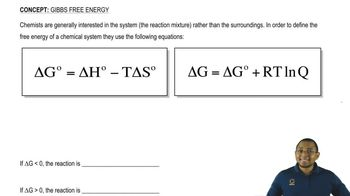06:13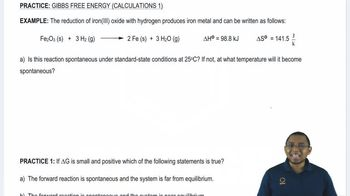06: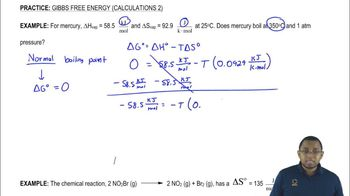03:26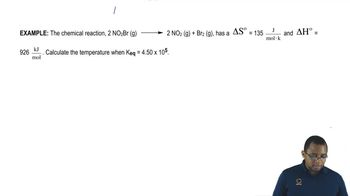04:08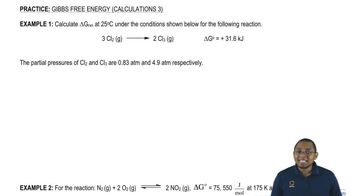02:37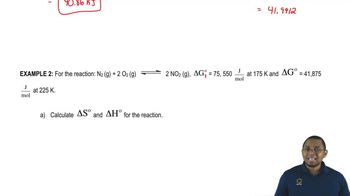04:01